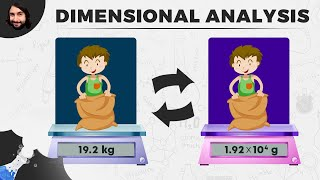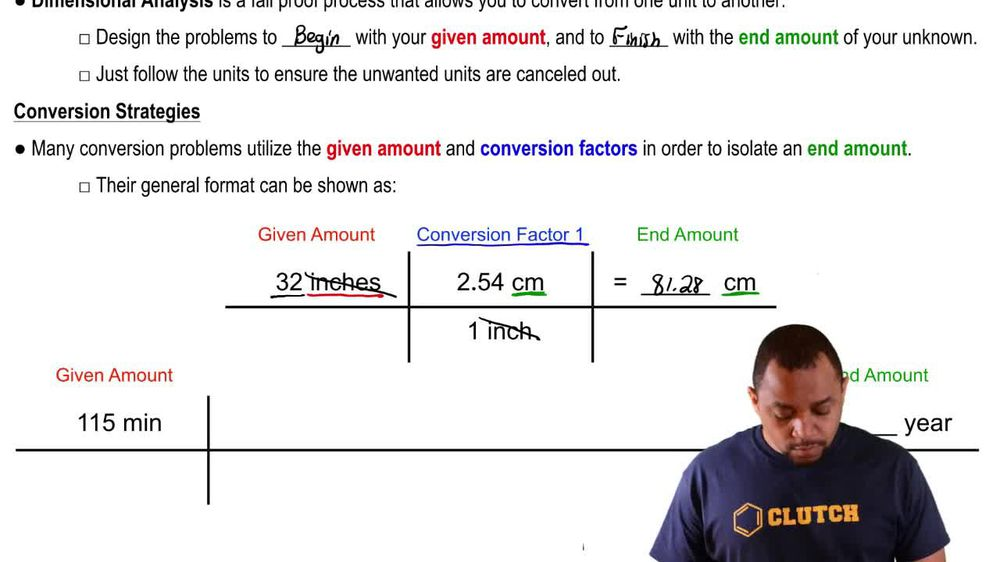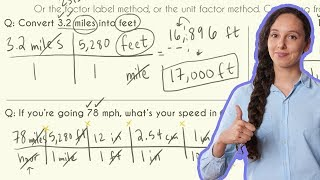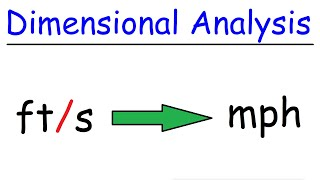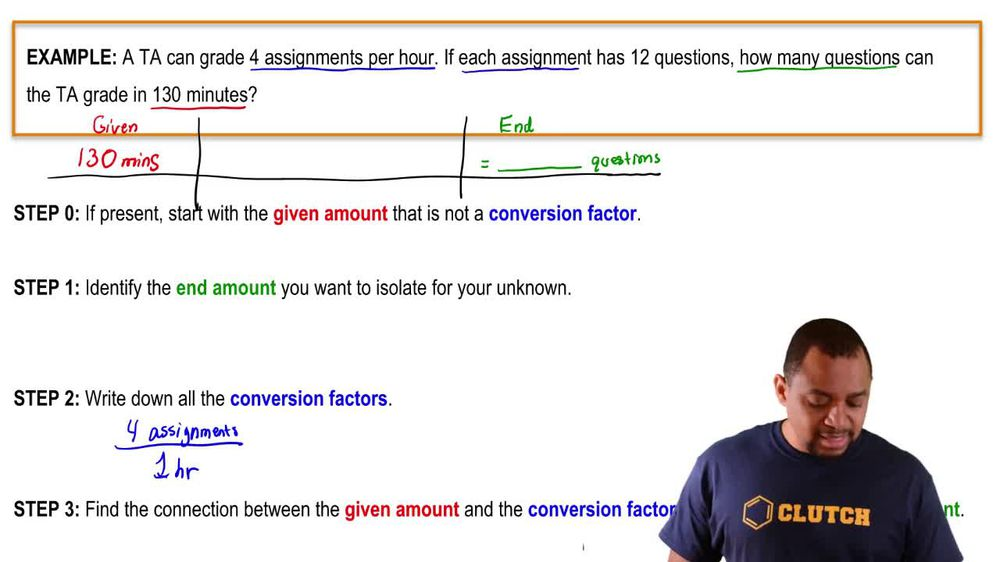Start typing, then use the up and down arrows to select an option from the list.
1. 1. Intro to General Chemistry2. Dimensional Analysis
Problem

# Perform each unit conversion. a. 1.4 in to mm b. 116 ft to cm c. 1845 kg to lb d. 815 yd to km

Relevant Solution2m
Play a video:
Hello everyone in this video, we're going to try to convert yards into millimeters. So this is essentially dimensional analysis. So how I think of dimensional analysis is kind of like a puzzle piece mathematic problem. So kind of like a fun math problem. So what we're gonna do is kind of use baby steps to convert the yards and two because I don't know what the direct conversion. So first I wanna know is that we're given this and we're trying to get into millimeters. Like I said, I do not know the direct conversion from yards into millimeters. So I'll do baby steps. Maybe we can try. So I know the comforting from yard into just meters And they're from m in two. So that's going to be my roadmap for my dimension analysis. So first starting off with the information that I am given. So I'm given the 0.780 Y. D. Was just abbreviation for yard. So we are here now we're trying to get into meters And I know for every one m It's going to be 1.094 yards. So multiplying this. So I've converted to meters. Now then I'm going from meters into millimeters. So for every one Millimeter is going to be 0.001 m. I'm just checking to see if my conversions are canceling out. We have yards canceling out with yards and meters canceling out with meters. You see here that the answer will have the millimeter unit and that's exactly what we want and the problem right there. Alright. So putting everything into my calculator, I'll get a value of 0.98 mila meter. So whenever you see a damage an analysis problem and you don't know the direct conversion, Not to worry, just do baby steps. The professor would never ask you to do it. And maybe just one step, there's definitely going to be baby steps and you kinda have to just work with what, you know, and maybe you can even do it in two steps, Maybe, you know, maybe maybe you can do it like five steps does not matter just as long as you get to the millimeters, then you're good to go, Alright, thank you so much for watching and this is going to be our final answer.